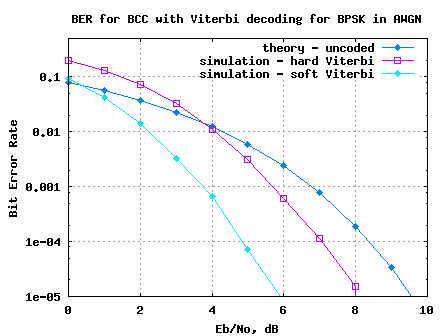- DSP log - http://www.dsplog.com -

Soft Input Viterbi decoder

Posted By Krishna Sankar On January 14, 2009 @ 10:41 am In Coding | 49 Comments

In two previous posts, we have discussed Convolutional Coding  and the associated hard decision Viterbi decoding . In this post lets extent Viterbi decoding algorithm to soft input decision scheme. The modulation used is BPSK and the channel is assumed to be AWGN alone.

## System Model

, where

is the modulated coded sequence taking values if the coded bit is 1 and if the coded bit is 0,

is the Additive White Gaussian Noise following the probability distribution function,

with mean and variance .

The conditional probability distribution function (PDF) of if the coded bit is 0 is,

.

Conditional probability distribution function (PDF) of if the coded bit is 1 is,

.

## Euclidean distance

In the hard decision Viterbi decoding, based on the location of the received coded symbol, the coded bit was estimated – if the received symbol is greater than zero, the received coded bit is 1; if the received symbol is less than or equal to zero, the received coded bit is 0.

In Soft decision decoding, rather than estimating the coded bit and finding the Hamming distance, the distance between the received symbol and the probable transmitted symbol is found out.

Euclidean distance if transmitted coded bit is 0 is,

.

Euclidean distance if transmitted coded bit is 1 is,
.

As the terms , , and are common in both the equations they can be ignored. The simplified Euclidean distance is,

and

.

As the Viterbi algorithm takes two received coded bits at a time for processing, we need to find the Euclidean distance from both the bits.

Summarizing, in Soft decision decoding, Euclidean distance is used instead of Hamming distance for branch metric and path metric computation.

Note:

For details on branch metric, path metric computation and trace back unit refer to the post on hard decision Viterbi decoding .

## Simulation Model

Octave/Matlab source code for computing the bit error rate for BPSK modulation in AWGN using the convolutional coding and soft decision Viterbi decoding is provided.

The simulation model performs the following:

(a) Generation of random BPSK modulated symbols +1’s and -1’s

(b) Convolutionally encode them using rate -1/2, generator polynomial [7,5] octal code

(c) Passing them through Additive White Gaussian Noise channel

(d) Received soft bits and hard bits are passed to Viterbi decoder

(e) Counting the number of errors from the output of Viterbi decoder

(f) Repeating the same for multiple Eb/No value.

(Warning: The simulation took around 5 hours in desktop to generate the plots)BER plot for BPSK with AWGN in soft decision Viterbi decoding

Figure: BER plot for BPSK with AWGN in soft decision Viterbi decoding

## Summary

1. When compared with hard decision decoding, soft decision decoding provides around 2dB of gain for bit error rate of .

2. In the current simulation model, soft bit are used with full precision for obtaining the BER curves. However in typical implementations, soft bits will be quantized to finite number of bits.

URL to article: http://www.dsplog.com/2009/01/14/soft-viterbi/

URLs in this post:

 Convolutional Coding: http://www.dsplog.com/2009/01/04/convolutional-code/

 Viterbi decoding: http://www.dsplog.com/2009/01/04/viterbi/

 Matlab/Octave script for computing BER for BPSK with AWGN in soft decision Viterbi decoding: http://www.dsplog.com/db-install/wp-content/uploads/2009/01/script_bpsk_ber_awgn_convlutional_code_soft_viterbi_decode.m